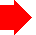## /* Neon Lights Text By JavaScript Kit (http://javascriptkit.com) For this script, TOS, and 100s more DHTML scripts, Visit http://www.dynamicdrive.com */ var message="TECHNICALSYMPOSIUM.COM \n An Education Website" var neonbasecolor="green" var neontextcolor="BLUE" var flashspeed=100 //in milliseconds ///No need to edit below this line///// var n=0 if (document.all||document.getElementById){ document.write('<font color="'+neonbasecolor+'">') for (m=0;m<message.length;m++) document.write('<span id="neonlight'+m+'">'+message.charAt(m)+'</span>') document.write('</font>') } else document.write(message) function crossref(number){ var crossobj=document.all? eval("document.all.neonlight"+number) : document.getElementById("neonlight"+number) return crossobj } function neon(){ //Change all letters to base color if (n==0){ for (m=0;m<message.length;m++) //eval("document.all.neonlight"+m).style.color=neonbasecolor crossref(m).style.color=neonbasecolor } //cycle through and change individual letters to neon color crossref(n).style.color=neontextcolor if (n<message.length-1) n++ else{ n=0 clearInterval(flashing) setTimeout("beginneon()",1500) return } } function beginneon(){ if (document.all||document.getElementById) flashing=setInterval("neon()",flashspeed) } beginneon()# Shortcut Formulae frequently used in solving Aptitude Question Papers

1. 1+2+3+4…. +n = n (n+1) / 2

2. 12+22+32…. +n2 = n (n+1)(2n+1) / 6

3. 13+23…+n3 = [n2(n+1)2] / 4

4. 1+3+5…. 2n-1= n2

5. Every prime number greater than 3 can be written using the formula (6k+1) or (6k-1)

6. Number of factors present in a number is given as (p+1)(q+1)(r+1)… Where N=(ap)+(bq)+(cr)… Where a, b, c are prime numbers and p, q, r are positive numbers In the same way sum of all factors=[(a(p+1) / (a-1)][(b(q+1) / (b-1)]…

7. If there is P volume of pure liquid initially in each operation and Q volume is taken out and replaced by Q volume of water then at the end of n such operations, the concentration k is given as K = [(P-Q) / P]n

8. If successive increase in percentage is given p%, q%, r% then effective % increase is given as [(100+p)/100][(100+q)/100][(100+r)/100]-1}*100

9. If an article is sold such that a article has a profit of p% on one and loss of p% on other then we have the net result to be loss and the loss percent is (p2) / 100Download Full Material(s)-Please Click here

# Technicalsymposium.com- All Information Contents Given Below-Click & Get All Details